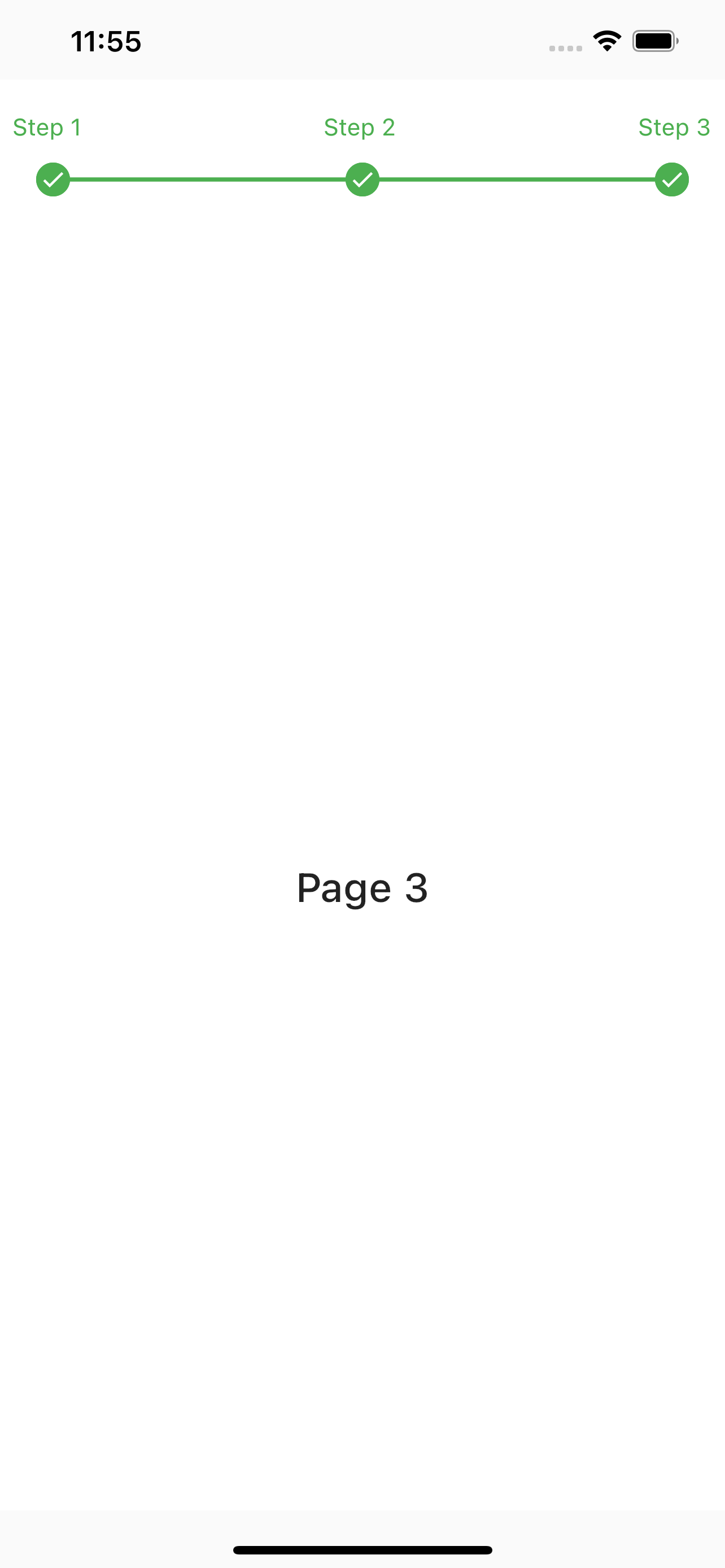# linear_step_indicator

## Install

In the `pubspec.yaml` of your flutter project, add the following dependency:

``````dependencies:
linear_step_indicator: ^1.0.0+3
``````

Import the package in your project:

``````import 'package:linear_step_indicator/linear_step_indicator.dart';
``````

## Getting Started

Examples:

``````FullLinearStepIndicator(
steps: 5,
lineHeight: 3.5,
activeNodeColor: Colors.brown,
inActiveNodeColor: const Color(0xffd1d5d8),
activeLineColor: Colors.brown,
inActiveLineColor: const Color(0xffd1d5d8),
controller: pageController,
labels: List<String>.generate(STEPS, (index) => "Step \${index + 1}"),
complete: () {
//typically, you'd want to put logic that returns true when all the steps
//are completed here
return Future.value(true);
},
)
````````````LinearStepIndicator(
steps: 3,
controller: _pageController,
labels: List<String>.generate(3, (index) => "Step \${index + 1}"),
complete: () {
//typically, you'd want to put logic that returns true when all the steps
//are completed here
return Future.value(true);
},
)
````````````StepIndicatorPageView(
steps: 3,
indicatorPosition: IndicatorPosition.top,
labels: List<String>.generate(3, (index) => "Step \${index + 1}"),
controller: _pageController,
complete: () {
//typically, you'd want to put logic that returns true when all the steps
//are completed here
return Future.value(true);
},
children: List<Widget>.generate(
3,
(index) => Container(
color: Color(0xffffffff),
child: Center(
child: Text(
"Page \${index + 1}",
style: TextStyle(
fontSize: 24,
fontWeight: FontWeight.w500,
),
),
),
),
),
)
``````## Contributions

Feel free to contribute to this project.

If you find a bug or want a feature, but don't know how to fix/implement it, please fill an issue.
If you fixed a bug or implemented a feature, please send a pull request.

## Libraries

linear_step_indicator